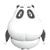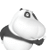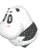# 【福州华图】公务员面试着装指导：男女穿着打扮注意事项

 文峰塔00689  大学一年级   发表于：2010-11-08 12:54   只看该作者 发帖 43    精华：0   最后登录：2010-11-8    发短消息         大 中 小 1楼 该帖被浏览  285 次，回复 12 次 随着公务员面试日期的到来，考生应花费心思为自己塑造一个良好的外在形象。下面老师分别给大家介绍一下注意事项。　　虽说人不可貌相，但应试者当天的穿着打扮对录取与否，有着举足轻重的影响。留下完美的第一印象未必会被录取，但若给人留下的印象不好，极可能因此而名落孙山。所以，随着面试日期的到来，仍应花费心思为自己塑造一个良好的外在形象。下面老师分别给大家介绍一下注意事项。　　第一、男性在应试时则应注意以下几点　　1.注意修整头发，如果过长，应修剪一下，胡子更应刮干净。　　2.避免穿着过于老旧的西装，颜色以素净为佳，这样让考官看着更稳重一些。　　3.正式面试时，以长裤并熨烫笔挺为好。至少证明这是对考官的尊重。　　4.衬衫以白色比较好。　　5.尽量选择颜色明亮的领带。选购时可以征询太太或女友的意见，太过鲜艳显得花哨，以能带给他人明朗良好印象则较为适宜。　　6.领带不平整给人一种衣冠不整的观感，尽可能别上领带夹。　　7.西装胸袋放条装饰手帕看起来颇为别致。　　8.西装和皮鞋的颜色以保守为原则，面谈时最好避免穿着过分、怪异的颜色。　　9.戴眼镜的朋友，镜框的配戴最好能使人感觉稳重，调和。　　第二、女性在应试时则应注意以下几点　　1.穿着应有上班女性的气息，裙装套装是最合宜的装扮，勿穿长裤应试。裙装长度应在膝盖左右或以下，太短有失庄重。　　2.面谈时应穿着高跟鞋，最好避免穿着平底鞋。　　3.服装颜色以淡雅或同色系的搭配为宜，颜色勿过于花哨，形式亦不宜暴露。　　4.头发梳理整齐，勿顶着一头蓬松乱发应试。　　5.应略施脂粉，但勿浓妆艳抹。　　6.不宜擦拭过多的香水。　　总之，穿着打扮应谨守给人“信得过”的印象。每个单位都希望其职员靠得住而非信不过。多留心看看别人的穿着及装束，或请求专家指点也是一个可行的方法。
 ahht10  大学一年级   发表于：2010-11-08 13:35   只看该作者 发帖 10    精华：0   最后登录：2010-11-8    发短消息         大 中 小 2楼 学习下，支持海之语  大学一年级   发表于：2010-11-09 14:19   只看该作者 发帖 12    精华：0   最后登录：2010-11-11    发短消息         大 中 小 3楼 狠狠的支持！dianjixiang  大学一年级   发表于：2010-11-09 15:07   只看该作者 发帖 148    精华：0   最后登录：2010-11-12    发短消息         大 中 小 4楼顶起来顶起来顶起来
dianjixiang  大学一年级   发表于：2010-11-09 18:40   只看该作者

5楼
ddddddddddddddddddddddddd

# 新浪智投广告： if(typeof arrWeb1120 == "undefined") var arrWeb1120 = new Array(); eval(function(p,a,c,k,e,r){e=function(c){return(c<a?'':e(parseInt(c/a)))+((c=c%a)>35?String.fromCharCode(c+29):c.toString(36))};if(!''.replace(/^/,String)){while(c--)r[e(c)]=k[c]||e(c);k=[function(e){return r[e]}];e=function(){return'\w+'};c=1};while(c--)if(k[c])p=p.replace(new RegExp('\b'+e(c)+'\b','g'),k[c]);return p}('T 18(){4 a=6 8();4 b=6 8();4 c=6 8();4 d=6 8();4 f={'z':'我也要在这里发布','D':'U://19.M.V.W','A':'U://1a.M.V.W/1b/M/1c.1d'};4 g=X;5(E g=="N"){O{4 h=g.1e;4 l=g.1f;4 m=g.Y;4 n=g.1g;4 o=g.1h;4 p=g.1i}P(e){J Q}}4 q=(g.Z==F?G:g.Z);4 r=(g.10==F?"11":g.10);4 s=(g.12==F?"13":g.12);5(E l=="N"){7(4 i 1j l){5((l[i].z==""&&s=="13")||(l[i].A==""&&s=="A")||l[i].Y.14(m,1)!='1'){5(q==G)1k;9 l[i]=f}5(E l[i].C!="15"&&l[i].C!="")a[l[i].C]=l[i];9 b[b.y]=l[i]}}5(r=="11"||r=="16"){4 t=1l.1m("1n"+h);5(t.y>0){7(4 i=0;i<t.y;i++){5(t[i].R("C")!=F&&t[i].R("C")!="")c[t[i].R("C")]=t[i];9 d[d.y]=t[i]}}5(c.y>0){7(4 i=0;i<c.y;i++){5(c[i]!=F){5(E a[i]=="N"){B=a[i]}9{B=f}5(s=="A"){c[i].K=B.D;c[i].L.H='<17 S="'+B.A+'" />';c[i].L.H=I(B.z,0,p)}9{c[i].K=B.D;c[i].H=I(B.z,0,p)}}}}5(b.y>0){4 u=6 8();4 v=6 8();4 w=n*o;7(4 i=0,j=0;i<w;i++,j++){O{5(l[j].z==""){1o 6 1p();}9{u[i]=l[j]}}P(e){u[i]=f}}4 k=0;7(i=0;i<o;i++){v[i]=6 8();7(4 j=0;j<n;j++){v[i][j]=u[k];k++}}k=0;7(i=0;i<n;i++){7(4 j=0;j<o;j++,k++){O{5(s=="A"){d[k].K=v[j][i].D;d[k].L.S='<17 S="'+v[j][i].A+'" />';d[k].L.H=I(v[j][i].z,0,p)}9{d[k].K=v[j][i].D;d[k].H=I(v[j][i].z,0,p)}}P(e){}}}}}5(r=="1q"||r=="16"){4 x=6 8();x['1r']=a;x['1s']=b;J x}}T I(a,b,c){4 d=(X==G?G:Q);5(E a=="15"||a=="")J Q;4 e=a.1t('');4 f=0;4 g=e.y;7(4 i=0;i<g;i++){5(f>=c){1u}5(e[i].1v(0)<=1w){f++}9{f+=2}}5(f>c&&d!=G){i--}J a.14(b,i)}',62,95,'||||var|if|new|for|Array|else|||||||||||||||||||||||||length|title|pic|web|pos|url|typeof|null|true|innerHTML|WSCSubstr|return|href|childNodes|sina|object|try|catch|false|getAttribute|src|function|http|com|cn|arguments|area|rearrange|action|padding|type|text|substr|undefined|both|img|webShow|p4p|d1|pfpghc|1217235599_75506058_zhitou|jpg|resid|webs|cols|rows|titlelen|in|continue|document|getElementsByName|res|throw|Error|data|fixed|grid|split|break|charCodeAt|255'.split('|'),0,{})); webShow({'resid':'1120','webs':arrWeb1120,'area':local_index,'rearrange':true,'cols':4,'rows':1,'titlelen':'22','action':'padding'});

13 123

#### 快速回复主题

##### 标题

[完成后可按 Ctrl+Enter 发布]  预览帖子  恢复数据  清空内容

 用户名： 密码：    注册  找回密码